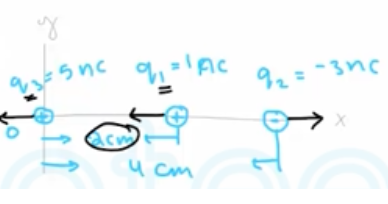Need Help?

Subscribe to Physics 2

###### \${selected_topic_name}
• Notes

Two point charges are located on the $x$ -axis of a coordinate system:
$q_{1}=1.0 \mathrm{nC}$ is at $x=+2.0 \mathrm{cm},$ and $q_{2}=-3.0 \mathrm{nC}$ is at $x=$$+4.0 \mathrm{cm} .$ What is the total electric force exerted by $q_{1}$ and $q_{2}$ on a
charge $q_{3}=5.0 \mathrm{nC}$ at $x=0 ?$$\overrightarrow{F_{3}}??$

${{\overrightarrow{F}}_{1 on 3}}??$

${{\overrightarrow{F}}_{2 on 3}}??$

$F_{1 on 3}=\frac{1}{4 \pi \varepsilon_{0}} \frac{\left|q, q_{3}\right|}{r_{13}^{2}}$

$F_{2 on}=\frac{1}{4 \pi \varepsilon_{0}} \frac{\left|q_{2} q_{3}\right|}{r_{23}^{2}}$

$F_{1 on 3} =9 * 10^{9} * \frac{|\left(1*10^{-9}\right)\left(5*10^{-9}\right)|}{(0.02)^{2}}$

$=1.12 * 10^{-4} N$

$=112 M_{N} =\vec{F}_{\text { i on } 3} =-(112 \mathrm{M_N}) \hat{\mathfrak{i}}$

$F_{2 on 3}=9 * 10^{9} * \frac{\left|\left(-3 * 10^{-9}\right)\left(5 * 10^{-9}\right)\right|}{(0.04)^{2}}$

$=84 M_ N=(84 M_ N \hat{i})$

$\vec{F}_{3}=\vec{F}_{1 on 3}+\vec{F}_{2 on 3}$

$=-112 \hat{\imath}+84 \hat{\imath}=-28 \hat{\imath}(M_N)$

Three point charges are arranged on a line. Charge
$q_{3}=+5.00 \mathrm{nC}$ and is at the origin. Charge $q_{2}=-3.00 \mathrm{nC}$ and is at $x=+4.00 \mathrm{cm} .$ Charge $q_{1}$ is at $x=+2.00 \mathrm{cm} .$ What is $q_{1}$
(magnitude and sign) if the net force on $q_{3}$ is zero?

$F_{2}={k} \frac{\left|q_{2} q_{3}\right|}{r_{2}^{2}}$

$=9 * 10^{9} * \frac{\left|-3 * 10^{-9} * 5 * 10^{-9}\right|}{\left(4 * 10^{-2}\right)^{2}}=$

$F_{2}=F_{1}=k \frac{|q_1 q_{3} |}{r_{1}^{2}}$

$F_{1}=F_{2} \longrightarrow k \frac{\left|q_{1}\right||q _ 3|}{r_{1}^{2}} =k \frac{|q_{2}| | q_{3} |}{r_{2}^{2}}$

$\longrightarrow \frac{|q_1 |}{r_{1}^{2}} \frac{\left|q_{2}\right|}{r_{2}^{2}} \rightarrow|q_1|= \left|q_{2}\right|\left(\frac{r_{1}}{r_{2}}\right)^{2}$

$= |-3 * 10^{-9}| (\frac{0.02}{0.04} )^{2}$

$∴ q_{1}=0.75 n C = 0.75 * 10^{-9} \mathrm{C}$